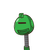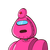# Practice Question 33.1 Find the marked price (M.P.) if the sale price (S.P.) is 9,250 and the discount is 7 1/2%​

Practice Question 3
3.1 Find the marked price (M.P.) if the sale price (S.P.) is 9,250 and the discount is 7 1/2%​

### 2 thoughts on “Practice Question 3<br />3.1 Find the marked price (M.P.) if the sale price (S.P.) is 9,250 and the discount is 7 1/2%​”

1.Jd8td8td7rdit7rsurzutzoyxoydoydoydoydoydoydigxogcoyxiydoyfoydoydoydoy

2.let the market price be x

selling price =market price-discount %/ 100 x mp

9250 =x-15/2×100 x ×

9250=200×\200 -15x

9250=185x/200

x=9250×200/ 185

x=1550000/185

x=10000

Step-by-step explanation: